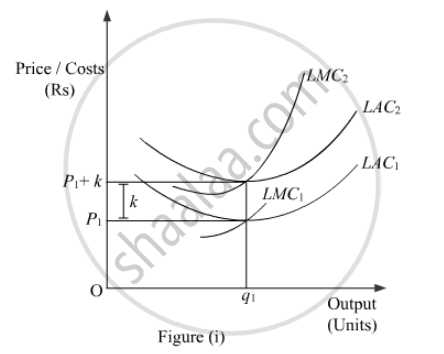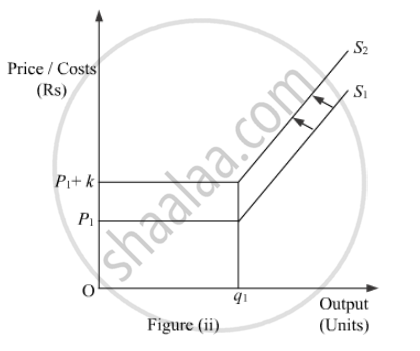# Define ‘Market-supply’. What is the effect on the supply of a good when Government imposes a tax on the production of that good? Explain. - Economics

#### Question

Define ‘Market-supply’. What is the effect on the supply of a good when Government imposes a tax on the production of that good? Explain.

#### Solution

Market supply refers to the total of quantities supplied by all the firms in the market at different price levels.

If, government imposes a tax on the production of a good then, this implies that the cost of production rises. Consequently, the firm will supply lesser units of output. The diagrammatic presentation of the effect on the supply of a good when government imposes a tax on the production of that good is as follows.In the diagram, LAC1 and LMC1 are the long run average cost curve and long run marginal cost curve respectively. In the lower panel we represent the supply curve which is the rising part of the LMC. Suppose, initially the firm faces price equal to OP1. Now, if the government imposes a unit tax of Rs ‘k’ per unit of output, then this will raise the cost of production as the firm need to pay an extra amount of Rs k on each unit of the output supplied. Consequently, the cost curves will shift leftwards (upwards) to LMC2 and LAC2. The magnitude of the shift in the cost curves is equal to Rs k. Now, as the supply curve is a rising part of LMC, so the supply curve in the figure (ii) will also shift leftward upwards from S1 to S2. That is, the firm will now supply lesser units of output due to imposition of tax.

Is there an error in this question or solution?

#### Video TutorialsVIEW ALL 

Define ‘Market-supply’. What is the effect on the supply of a good when Government imposes a tax on the production of that good? Explain. Concept: Market Supply.